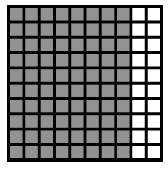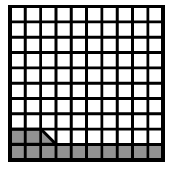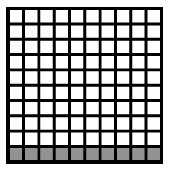### Home > CCAA > Chapter 1 CC2: Unit 1 > Lesson CC2: 1.2.1 > Problem1-60

1-60.

FRACTIONS AND PERCENTS

Marianna represented several percents as portions of $100$ in the pictures below.

1.1.1.1. Write the percent represented in each picture.

A percent refers to an amount out of $100$.

Count how many shaded squares there are out of $100$.

1. has $80$ shaded squares.

2. has $12$ and a half shaded squares.

3. has $10$ shaded squares.

1. $80\%$

2. $12.5\%$

3. Now try this on your own.

2. Write the portion represented in each picture as a fraction in at least two different ways.

Based on part (a), what is the number of shaded squares over the total number of squares?

Can you reduce these fractions?